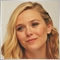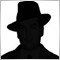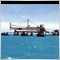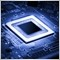• Overview
• Reviews (7)

# ImpAC

Indicator Accelerator/Decelerator Oscillator (AC) is calculated using median price and simple moving averages with periods 5/34 and 5.

This modification allows calculating indicator on the basis of any combination of 4 basic prices: Close, Open, High, and Low. Also it allows selecting periods and methods of moving average: simple (SMA), exponential (EMA), smoothed (SMMA), linear weighted (LWMA), double exponential (DEMA), triple exponential (TEMA).

Input parameters:

• Fast period - period of fast moving average;
• Slow period - period of slow moving average;
• AC period - period of fast moving average for AC;
• Moving average mode - averaging method: Simple (SMA), Exponential (EMA), Smoothed (SMMA), Linear Weighted (LWMA), Double Exponential (DEMA), Triple Exponential (TEMA).
• Close - weight coefficient of the CLOSE price for calculation of the individual price;
• Open - weight coefficient of the OPEN price for calculation of the individual price;
• High - weight coefficient of the HIGH price for calculation of the individual price;
• Low - weight coefficient of the LOW price for calculation of the individual price.

Example 1. Calculate indicator by OPEN price: Close=0, Open=1, High=0, Low=0.
Example 2. Calculate indicator by WEIGHTED price: Close=2, Open=0, High=1, Low=1.
Example 3. Calculate indicator by individual price constant: Close=6, Open=7, High=1, Low=3. Price=(6*Close + 7*Open + 1*High + 3*Low)/17.424
2019.11.04 17:49

interesting16477
Nikolay Gaylis 2019.01.14 08:23

User didn't leave any comment to the rating12010
2018.02.01 17:30

User didn't leave any comment to the rating5667
2017.11.09 14:56

User didn't leave any comment to the rating6158
2017.07.21 18:31

User didn't leave any comment to the rating7173
2016.03.12 21:56

User didn't leave any comment to the rating7826
2014.04.08 12:31

User didn't leave any comment to the rating